2019-11-19 16:30:01 niaolianjiulin 阅读数 39
• ###### 5天搞定深度学习框架-Caffe入门系列

10722 人正在学习 去看看 覃秉丰

# caffe读取图像

1、设定图片的shape格式为网络data层格式
transformer = caffe.io.Transformer({'data': net.blobs['data'].data.shape})

2、改变维度顺序，由原始图片维度(h, w, c)变为(c, h, w)
transformer.set_transpose('data', (2,0,1))

3、减去图像均值,注意要先将binaryproto格式均值文件转换为npy格式

transformer.set_mean('data', [b_v, g_v, r_v] )

4、缩放到[0，255]之间
transformer.set_raw_scale('data', 255)

5、交换通道，将C通道由RGB变为BGR
transformer.set_channel_swap('data', (2,1,0))

6、加载图片

7、执行上面设置的图片预处理操作，并将图片载入到blob中
net.blobs['data'].data[...] = transformer.preprocess('data', im)


# opencv读图

（1）数据格式是BGR
（2）数值范围0~255

2、

3、注意区分2个变换：
（1）transformer.set_transpose(‘data’, (2,0,1)) 这个是将HWC->CHW，不管是caffe.io读图，还是opencv读图，这步操作都是需要的。
（2）transformer.set_channel_swap(‘data’, (2,1,0))这个是将C通道的RGB->BGR。该操作只是在caffe.io读图时需要做下。opencv读图时本身就已经是BGR了。

2018-01-05 15:31:36 oJiMoDeYe12345 阅读数 3010
• ###### 5天搞定深度学习框架-Caffe入门系列

10722 人正在学习 去看看 覃秉丰

# Caffe2 - 图像加载与预处理

sudo pip install scikit-image scipy matplotlib
import skimage
import skimage.io as io
import skimage.transform
import sys
import numpy as np
import math
import matplotlib.pyplot as plt
import matplotlib.image as mpimg
print("Required modules imported.")

Caffe 使用的是 OpenCV 的 Blue-Green-Red (BGR)，而不是通用的 Red-Green-Blue (RGB).

Caffe2 也使用 BGR.

IMAGE_File = 'https://timgsa.baidu.com/timg?image&quality=80&size=b9999_10000&sec=1515142841038&di=56e548070fdf3ed09c1dc95a0ee2204f&imgtype=0&src=http%3A%2F%2Fimg6.ph.126.net%2FEfFAh8rDic7haOAZAQ83ag%3D%3D%2F2497527468371353486.jpg'

# show the original image
plt.figure()
plt.subplot(1,2,1)
plt.imshow(img)
plt.axis('on')
plt.title('Original image = RGB')

# show the image in BGR - just doing RGB->BGR temporarily for display
imgBGR = img[:, :, (2, 1, 0)]
#plt.figure()
plt.subplot(1,2,2)
plt.imshow(imgBGR)
plt.axis('on')
plt.title('OpenCV, Caffe2 = BGR')
plt.show()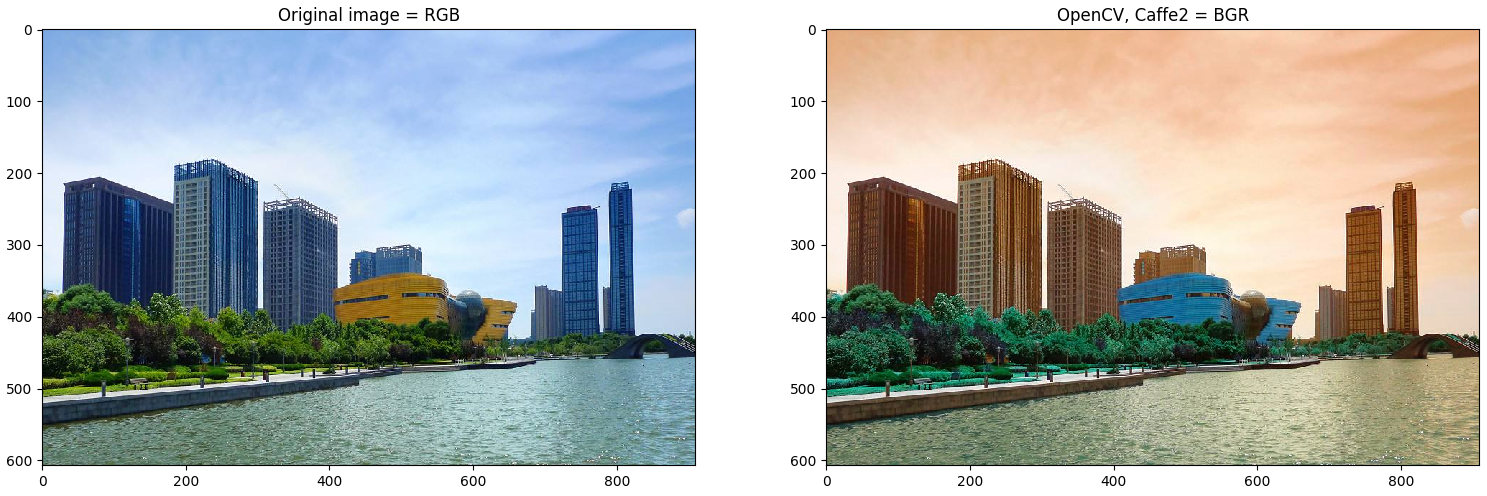Caffe 的 CHW 是指：

• H: Height
• W: Width
• C: Channel (as in color)

GPU 采用 CHW；而 CPU 往往是 HWC.

cuDNN 使用来对 GPUs 加速计算的工具，只支持 CHW.

## 2. Image Mirror 和 Rotate

# Flip， Mirror
imgMirror = np.fliplr(img)
plt.figure()
plt.subplot(1,2,1)
plt.imshow(imgMirror)
plt.axis('off')
plt.title('Mirror image')

# Rotate
imgRotated = np.rot90(img)
plt.subplot(1,2,2)
plt.imshow(imgRotated)
plt.axis('off')
plt.title('Rotated image')
plt.show()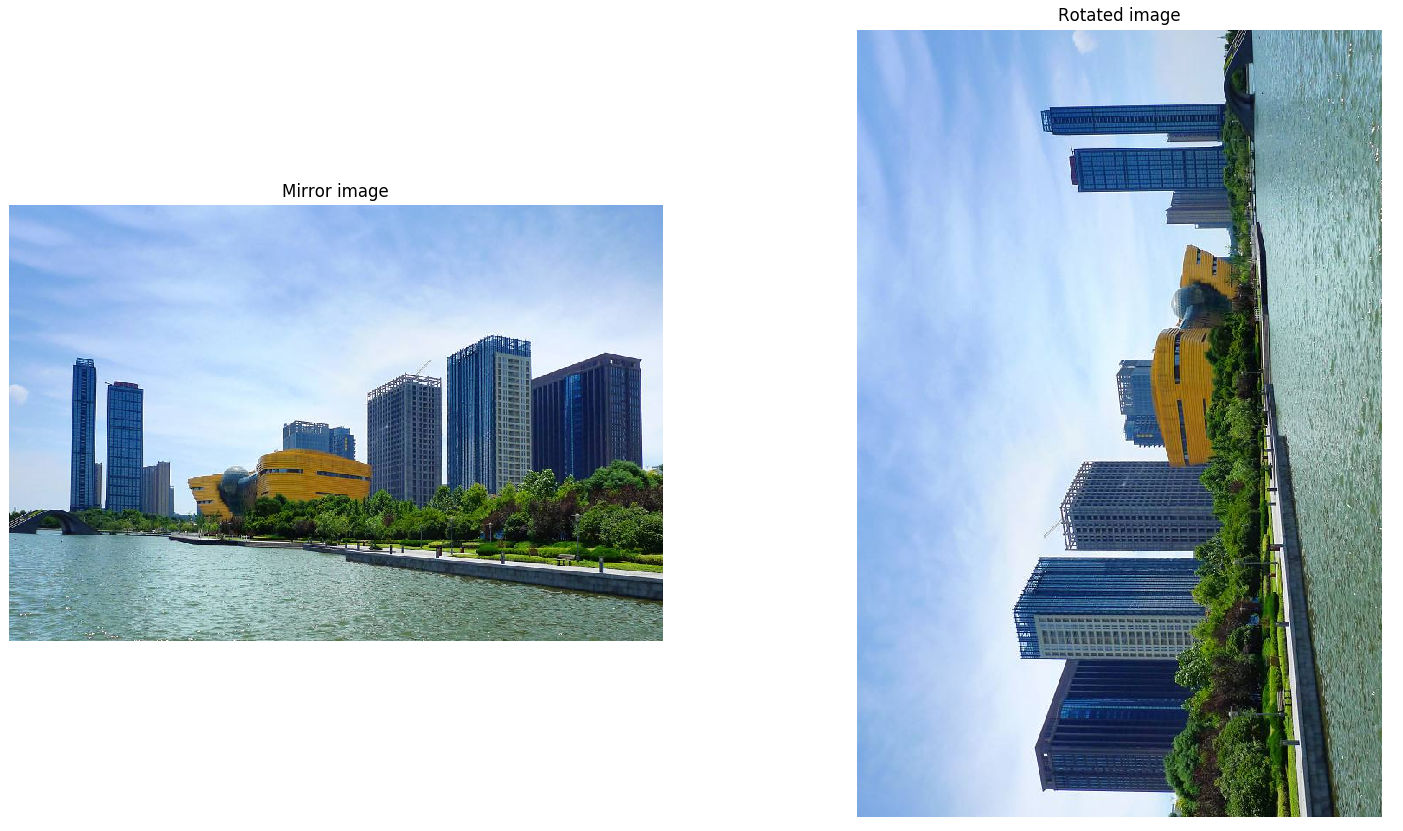## 3. Image Resize

resize 是图像预处理很重要的一部分.

Caffe2 要求图片是方形(square)的，需要 resize 到标准的 height 和 width. 这里将图片 resize 为 $256×256$$256×256$，然后 crop 出 $224×224$$224×224$ 的尺寸大小. 即为网络输入的图片 input_height 和 input_width.

input_height, input_width = 224, 224
print("Model's input shape is %dx%d") % (input_height, input_width)
img256 = skimage.transform.resize(img, (256, 256))
plt.figure()
plt.imshow(img256)
plt.axis('on')
plt.title('Resized image to 256x256')
print("New image shape:" + str(img256.shape))
plt.figure()

Model’s input shape is 224x224
New image shape:(256, 256, 3)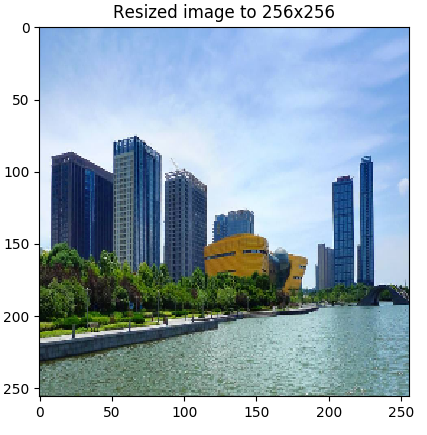## 4. Image Rescale

print("Original image shape:" + str(img.shape) + " and remember it should be in H, W, C!")
print("Model's input shape is %dx%d") % (input_height, input_width)
aspect = img.shape/float(img.shape)
print("Orginal aspect ratio: " + str(aspect))
if(aspect>1):
# 宽图片 - width 较大
res = int(aspect * input_height)
imgScaled = skimage.transform.resize(img, (input_height, res))
if(aspect<1):
# 长图片 - height 较大
res = int(input_width/aspect)
imgScaled = skimage.transform.resize(img, (res, input_width))
if(aspect == 1):
imgScaled = skimage.transform.resize(img, (input_height, input_width))
plt.figure()
plt.imshow(imgScaled)
plt.axis('on')
plt.title('Rescaled image')
print("New image shape:" + str(imgScaled.shape) + " in HWC")
plt.show()

Original image shape:(607, 910, 3) and remember it should be in H, W, C!
Model’s input shape is 224x224
Orginal aspect ratio: 1.49917627677
New image shape:(224, 335, 3) in HWC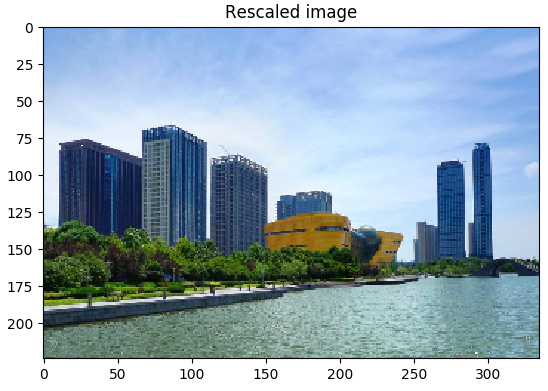## 5. Image Crop

# Center crop on the original
print("Original image shape:" + str(img.shape) + " and remember it should be in H, W, C!")
def crop_center(img,cropx,cropy):
y,x,c = img.shape
startx = x//2-(cropx//2)
starty = y//2-(cropy//2)
return img[starty:starty+cropy,startx:startx+cropx]

plt.figure()
# Original image
imgCenter = crop_center(img,224,224)
plt.subplot(1,3,1)
plt.imshow(imgCenter)
plt.axis('on')
plt.title('Original')

# Now let's see what this does on the distorted image
img256Center = crop_center(img256,224,224)
plt.subplot(1,3,2)
plt.imshow(img256Center)
plt.axis('on')
plt.title('Squeezed')

# Scaled image
imgScaledCenter = crop_center(imgScaled,224,224)
plt.subplot(1,3,3)
plt.imshow(imgScaledCenter)
plt.axis('on')
plt.title('Scaled')
plt.show()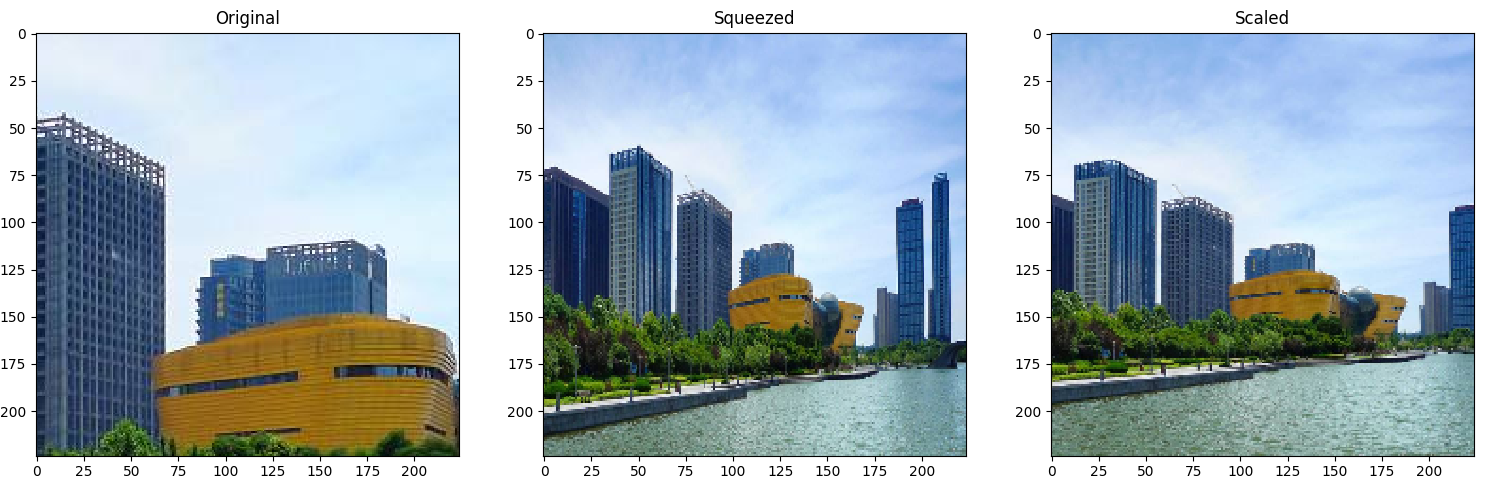## 6. Image Upscale

imgSmall128 = skimage.transform.resize(img, (128, 128))
print "Assume original image shape: ", imgSmall128.shape
imgBig224 = skimage.transform.resize(imgSmall128, (224, 224))
print "Upscaled image shape: ", imgBig224.shape
# Plot original
plt.figure()
plt.subplot(1, 2, 1)
plt.imshow(imgSmall128)
plt.axis('on')
plt.title('128x128')
# Plot upscaled
plt.subplot(1, 2, 2)
plt.imshow(imgBig224)
plt.axis('on')
plt.title('224x224')
plt.show()

Assume original image shape: (128, 128, 3)
Upscaled image shape: (224, 224, 3)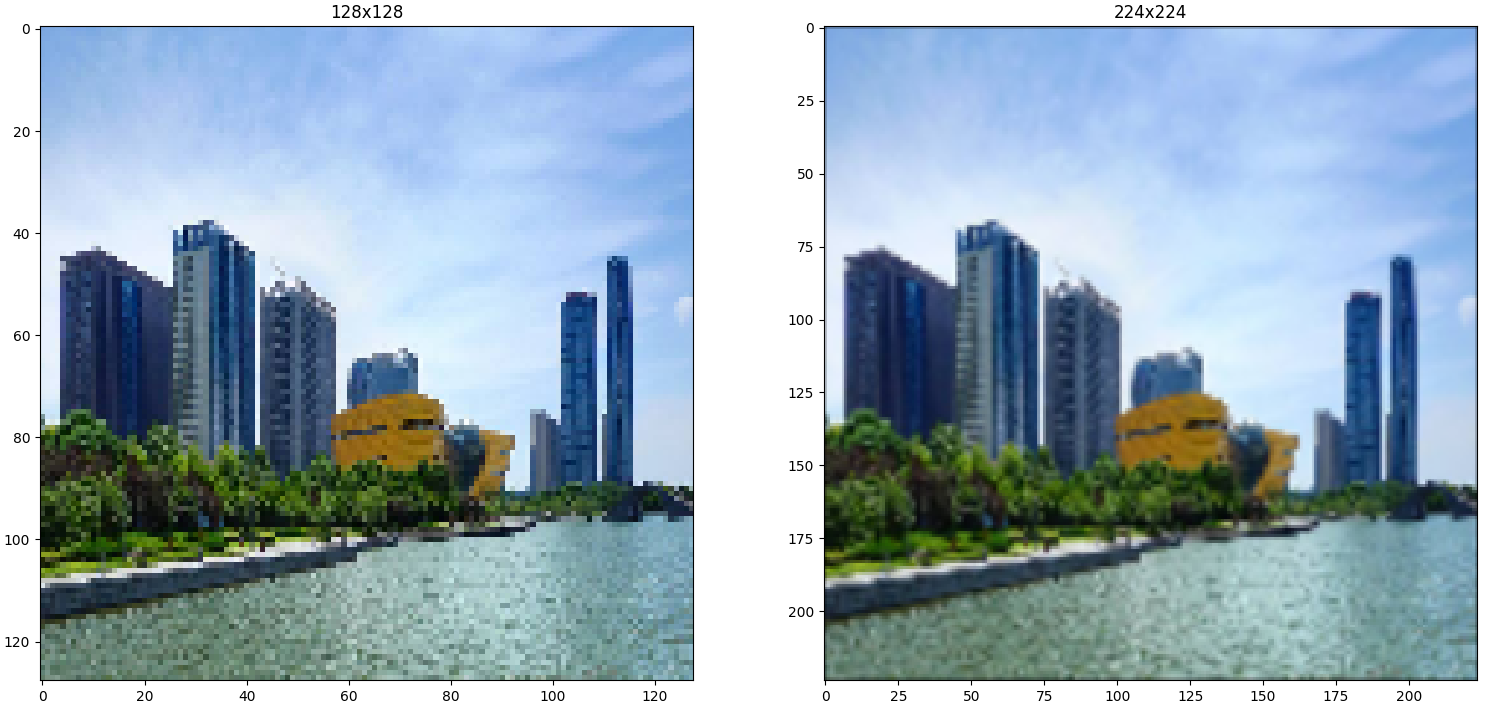imgSmallSlice = crop_center(imgSmall128, 128, 56)
# Plot original
plt.figure()
plt.subplot(1, 3, 1)
plt.imshow(imgSmall128)
plt.axis('on')
plt.title('Original')
# Plot slice
plt.subplot(1, 3, 2)
plt.imshow(imgSmallSlice)
plt.axis('on')
plt.title('128x56')
# Upscale?
print "Slice image shape: ", imgSmallSlice.shape
imgBigg224 = skimage.transform.resize(imgSmallSlice, (224, 224))
print "Upscaled slice image shape: ", imgBigg224.shape
# Plot upscaled
plt.subplot(1, 3, 3)
plt.imshow(imgBigg224)
plt.axis('on')
plt.title('224x224')
plt.show()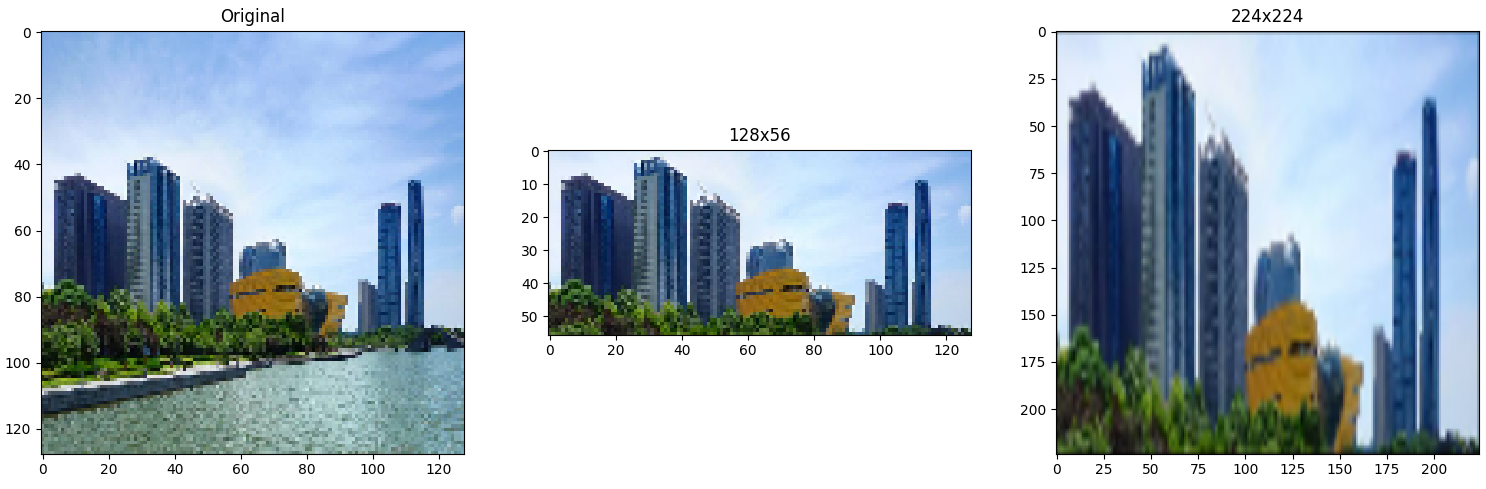## 7. Image Preprocessing

imgCropped = crop_center(imgScaled,224,224)
print "Image shape before HWC --> CHW conversion: ", imgCropped.shape
# HWC -> CHW
imgCropped = imgCropped.swapaxes(1, 2).swapaxes(0, 1)
print "Image shape after HWC --> CHW conversion: ", imgCropped.shape

plt.figure()
for i in range(3):
plt.subplot(1, 3, i+1)
plt.imshow(imgCropped[i])
plt.axis('off')
plt.title('RGB channel %d' % (i+1))

# RGB -> BGR
imgCropped = imgCropped[(2, 1, 0), :, :]
print "Image shape after BGR conversion: ", imgCropped.shape

# Subtract the mean image
# skimage loads image in the [0, 1] range so we multiply the pixel values first to get them into [0, 255].
# mean_file = os.path.join(CAFFE_ROOT, 'python/caffe/imagenet/ilsvrc_2012_mean.npy')
# img = img * 255 - mean[:, np.newaxis, np.newaxis]

plt.figure()
for i in range(3):
plt.subplot(1, 3, i+1)
plt.imshow(imgCropped[i])
plt.axis('off')
plt.title('BGR channel %d' % (i+1))
# Batch
# Feed in multiple images
# Make sure image is of type np.float32
imgCropped = imgCropped[np.newaxis, :, :, :].astype(np.float32)
print 'Final input shape is:', imgCropped.shape
plt.show()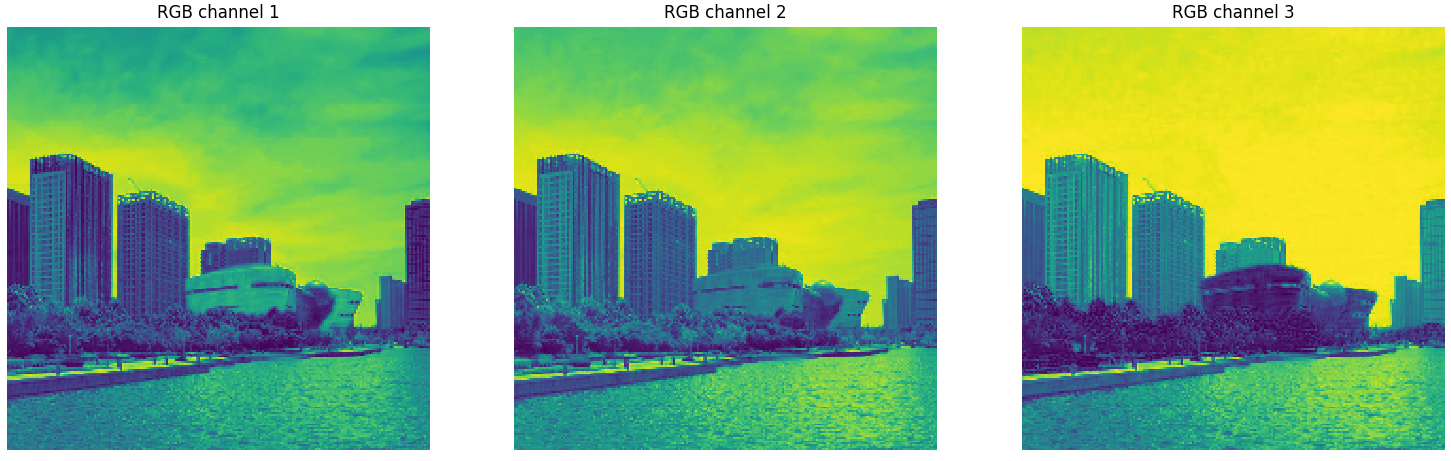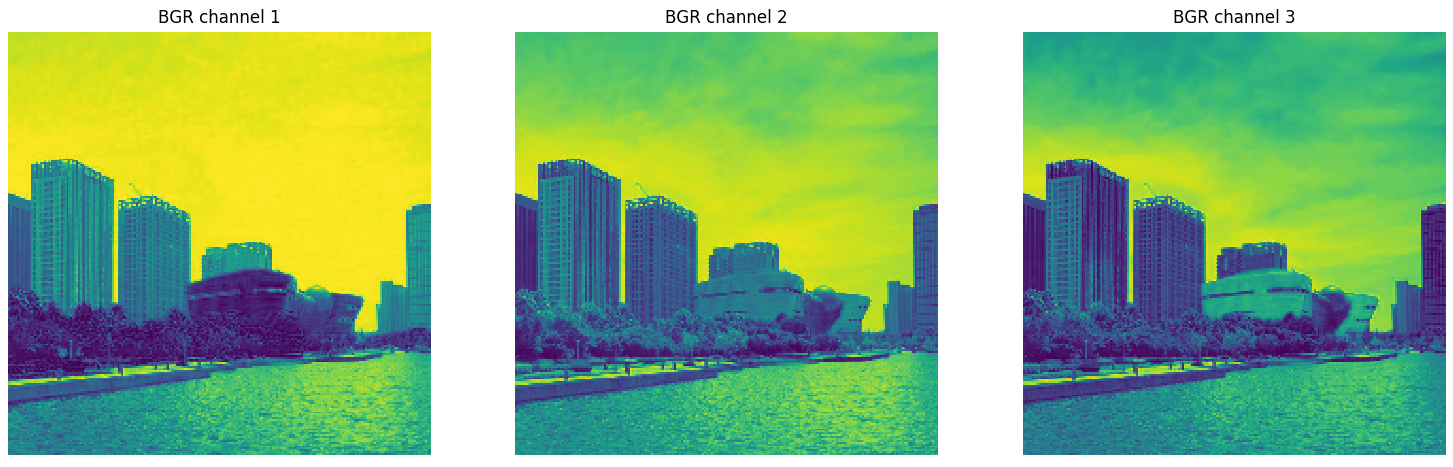2017-07-11 16:33:01 fuwenyan 阅读数 5318
• ###### 5天搞定深度学习框架-Caffe入门系列

10722 人正在学习 去看看 覃秉丰

MATLAB中RGB图像是存储在H*W*CH的三维矩阵中，其中H表示hight（即rows），W表示width（即cols），CH即channel。

caffe使用的图像是BGR格式，且矩阵维度为W*H*CH。

Img = permute(Img, [2,1,3]);%flip width and height
Img = Img(:,:,[3,2,1]);%permute channels from RGB to BGR

2016-06-30 22:34:58 dsif1995 阅读数 11807
• ###### 5天搞定深度学习框架-Caffe入门系列

10722 人正在学习 去看看 覃秉丰

convert_imageset是Caffe提供的图像转换工具，用于将训练图像集和验证图像集转换成Caffe方便处理的lmdb或leveldb的数据集。

该工具通过命令行方式使用，命令行的格式如下：
convert_imageset [FLAGS] ROOTFOLDER/ LISTFILE DB_NAME
其中DB_NAME后面还可以跟一些可选的参数设置，具体有哪些可选的参数参见“可选参数设置部分”
其中ROOTFOLDER为图像集的根目录
LISTFILE 为一个文件的路径，该文件中记录了图像集中的各图样的路径和相应的标注
DB_NAME为要生成的数据库的名字

举个例子：
convert_imageset ImgSetRootDir/ ImgFileList.txt imgSet.lmdb
其中ImgFileList.txt（也即LISTFILE）的没一行给出一个图像的信息，如：subfolder1/file1.JPEG 7
其中subfolder1/file1.JPEG为图像路径，7为该图像的类别，并且中间空一个空格

gray：bool类型，默认为false，如果设置为true，则代表将图像当做灰度图像来处理，否则当做彩色图像来处理
shuffle：bool类型，默认为false，如果设置为true，则代表将图像集中的图像的顺序随机打乱
backend：string类型，可取的值的集合为{"lmdb", "leveldb"}，默认为"lmdb"，代表采用何种形式来存储转换后的数据
resize_width：int32的类型，默认值为0，如果为非0值，则代表图像的宽度将被resize成resize_width
resize_height：int32的类型，默认值为0，如果为非0值，则代表图像的高度将被resize成resize_height
check_size：bool类型，默认值为false，如果该值为true，则在处理数据的时候将检查每一条数据的大小是否相同
encoded：bool类型，默认值为false，如果为true，代表将存储编码后的图像，具体采用的编码方式由参数encode_type指定
encode_type：string类型，默认值为""，用于指定用何种编码方式存储编码后的图像，取值为编码方式的后缀（如'png','jpg',...)

带参数的命令：
convert_imageset ImgSetRootDir/ ImgFileList.txt imgSet.lmdb --gray=true --resize_width=160 --resize_height=160

2016-11-15 21:07:52 xx_0401 阅读数 1117
• ###### 5天搞定深度学习框架-Caffe入门系列

10722 人正在学习 去看看 覃秉丰

没有过多的甄别的选择了caffe框架来实现一项关于脑部MR图像的分割任务，遇到了不少问题。win7下安装了caffe，然后编写protxt文档，处理数据集。卡在了处理数据集这个上面很久，因为caffe中需要将数据写成lmdb或者leveldb格式，lmdb和leveldb对应的是两种类型的数据库，其中lmdb是基于二叉树的数据库，leveldb是key-value型数据库。都具有简单、读写高效的特性。
一是修改convert_imageset.cpp，使得它能够读入raw格式数据，二是继续调研caffe是否允许其它方式导入数据，是否存在其它数据导入接口的可能性，三是换框架，经查证keras能够直接支持raw的处理，这里又不得不抛出一个新问题给小白的我了，不同框架的选择对于应用型研究是否最简单易于实现的便是最佳呢？不同框架会对本身网络实现的性能有什么影响呢？